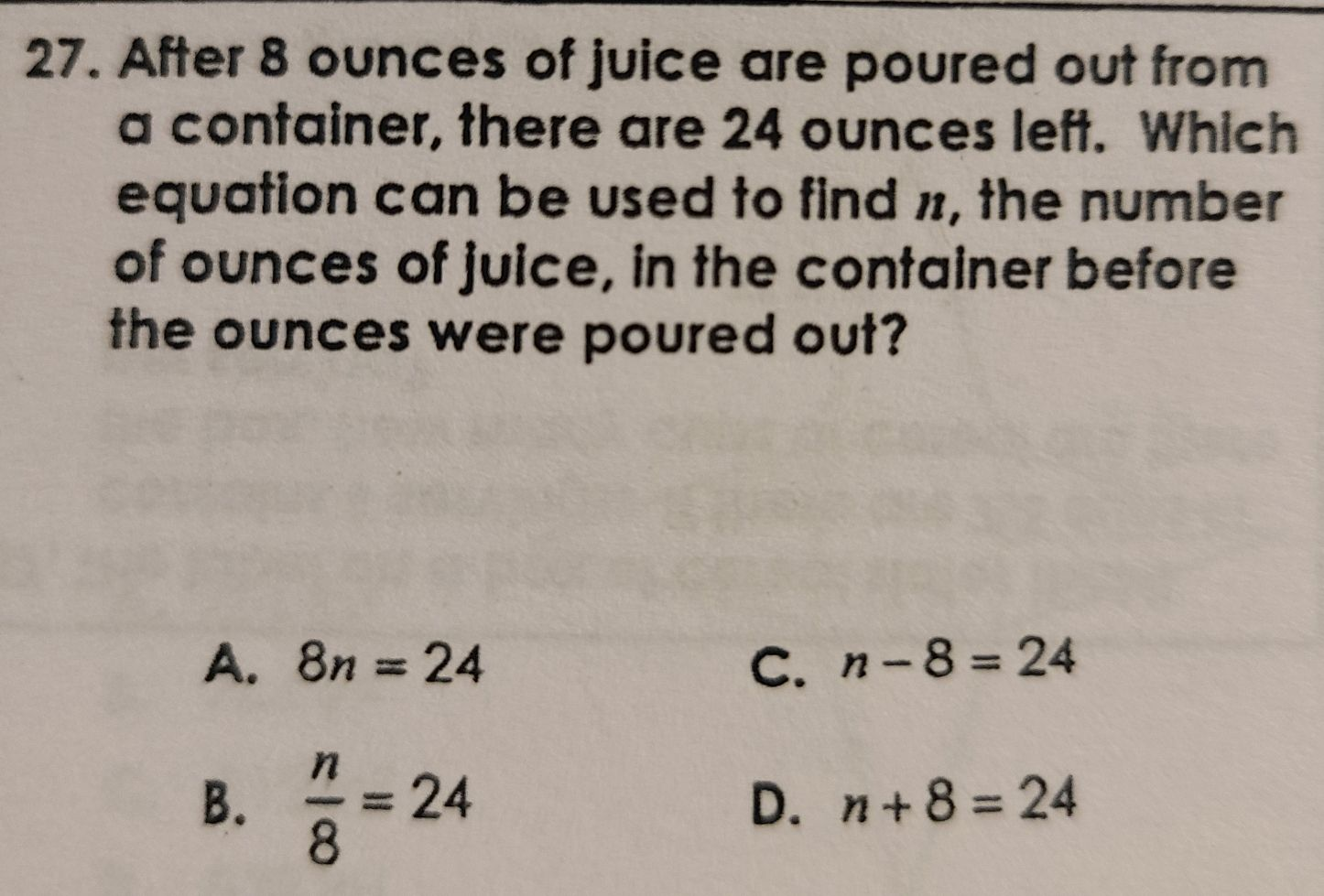### ¿Todavía tienes preguntas de matemáticas?

Pregunte a nuestros tutores expertos
Algebra
Pregunta27. After $$8$$ ounces of juice are poured out from a container, there are $$24$$ ounces left. Which equation can be used to find $$n$$ , the number of ounces of julce, in the container before the ounces were poured out?

A. $$8 n = 24$$ C. $$n - 8 = 24$$

B. $$\frac { n } { 8 } = 24$$ D. $$n + 8 = 24$$